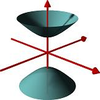#### You may also like### A Close Match

Can you massage the parameters of these curves to make them match as closely as possible?### Prime Counter

A short challenge concerning prime numbers.### The Right Volume

Can you rotate a curve to make a volume of 1?

# Common Divisor

### Why do this problem?

This problem can be used to introduce students to number theory and to how we can prove some divisibility facts. They offer an intriguing context for applying standard algebraic techniques.

### Possible approach

This activity featured in an NRICH Secondary webinar in March 2021.

For these problems it is a good idea for students to start by working through some numerical examples (perhaps with a calculator for the later ones!).

This article on divisibility tests might be useful, and you could use Dozens as a warm up task.

Students could be asked how they can tell that a number is divisible by 6.  For example, which of the following must be divisible by 6 (without actually dividing it!):

$$2574, 8961, 5972, 8981$$

They can also be asked to create a 6 digit number which must be divisible by 6. They can then be asked which numbers we need to be able to divide $n^3 - n$ by to show that it is divisible by 6. Factorising and considering the factors is helpful.

For the second two problems, students can be encouraged to write the common divisor as a product of prime numbers.

See also the key ideas section below.

### Key ideas

Problem 1:  $n^3-n$
Students can find the first few values of $n^3 - n$ and see what they notice. They may possibly spot that they are even before they spot that they are all multiples of 6. Students could be asked what being divisible by 6 means. For example, can they decide whether 1428 is divisible by 6 without dividing it? (They may need reminding of how they can show a number is divisible by 3 by considering the digit sum of the number).

They could think about breaking 6 down into a product of prime factors. Students may need to be given a nudge into considering factorisation, but once they have done so they could be asked what the notice about the individual parts of this factorisation. They could substitute some values into $(n-1)n(n+1)$ first if they are unsure. They could then be asked what they know about three consecutive numbers, in particular what we know about multiples of 2 and 3.

Another approach is to consider the cases $n=2k$ and $n=2k+1$ ($n$ is even and odd) to show that the expression $n^3 - n$ has a factor of 2, and $n=3k$, $n=3k+1$, $n=3k+2$ ($n$ is a multiple of 3, or one more or two more than a multiple of 3) to show that $n^3 - n$ has a factor of 3. This is easier to do if they consider the factorised version $(n-1)n(n+1)$.

Problem 2:  $n^5-n^3$
The second problem draws on the ideas of the first. This time the expressions can be divided by 24 so students need to show that it can be divided by 3 and by $2^3$. The factor of 3 can be argued from the fact that we have a product of three consecutive numbers, but the factor of $2^3$ is a little trickier. One option is to consider $n$ even and $n$ odd separately.

Problem 3:  $n^5-n$
For the third problem the factorisation is a little trickier and students might need a prompt to factorise completely. The factors of 2 and 3 follow in a similar way to before. Following on from the previous ideas, students could consider the cases $n=5k$, $n=5k+1$ etc. to show that the expression always has a factor of 5.

Problem 4:  $2^{2n}-1$
For the last problem, factorising gives two expressions which differ by 2. Inserting the in-between number and considering the prime factors of this will help to solve the problem.

### Possible support

Students could start by working on Take Three from Five where they are introduced to the fact that numbers can be written as a multiple of 3, 1 more than a multiple of 3 or 2 more than a multiple of 3.

Students could start by considering $n^2 + n$ and showing that this is always even. The could also show that $n(n+1)(n+2)$ always has a factor of three, possibly by considering $n=3k$, $n=3k+1$ and $n=3k+2$. The fact that in any three consecutive numbers there is always a multiple of three is useful in all of the four problems.

For students who find it difficult to see that any number can be written as one of $3k, 3k+1, 3k+2$ they could consider the three following sequences: $$1, 4, 7, 10, ...$$ $$2, 5, 8, 11, ...$$ $$3, 6, 9, 12, ...$$

Finding the formulae for these three sequences shows that any number has one of the three different forms.